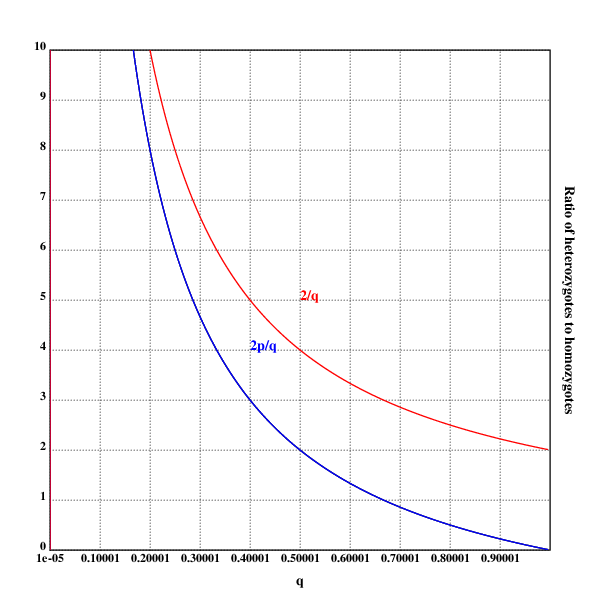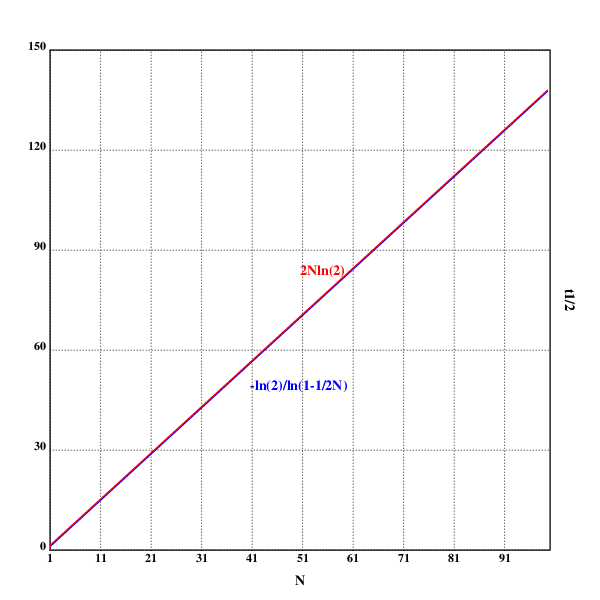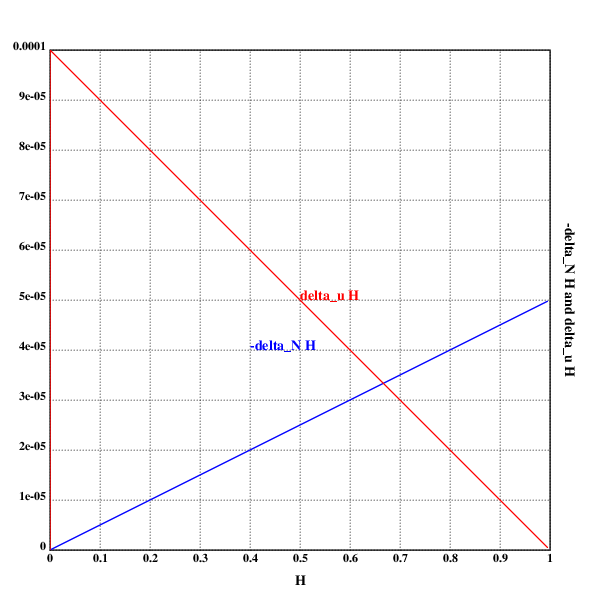## home

author: niplav, created: 2022-01-25, modified: 2022-02-23, language: english, status: in progress, importance: 2, confidence: likely

Some solutions to exercises in the book “Population Genetics” by John H. Gillespie. I did not simply copy out the solutions at the end of each chapter, and sometimes didn't even check the solutions against my own. Therefore, these might be faulty.

# Solutions to “Population Genetics”

## Chapter 1

### Problem 1.1

For three it would be $A_1A_1A_1, A_1A_1A_2, A_1A_2A_2, A_1A_2A_3, A_2A_2A_2, A_2A_2A_3, A_3A_3A_3$, with 7 different genotypes.

For four it would be 32 different genotypes:

$A_1 A_1 A_1 A_1, A_1 A_1 A_1 A_2, A_1 A_1 A_1 A_3, A_1 A_1 A_1 A_4, A_1 A_1 A_2 A_2$, $A_1 A_1 A_2 A_3, A_1 A_1 A_2 A_4, A_1 A_1 A_3 A_3, A_1 A_1 A_3 A_4, A_1 A_1 A_4 A_4$, $A_1 A_2 A_2 A_2, A_1 A_2 A_2 A_3, A_1 A_2 A_2 A_4, A_1 A_2 A_3 A_4, A_1 A_2 A_4 A_4$, $A_1 A_3 A_4 A_4, A_1 A_4 A_4 A_4, A_2 A_2 A_2 A_2, A_2 A_2 A_2 A_3, A_2 A_2 A_2 A_4$, $A_2 A_2 A_3 A_3, A_2 A_2 A_3 A_4, A_2 A_2 A_4 A_4, A_2 A_3 A_3 A_3, A_2 A_3 A_3 A_4$, $A_2 A_3 A_4 A_4, A_2 A_4 A_4 A_4, A_3 A_3 A_3 A_3, A_3 A_3 A_3 A_4, A_3 A_3 A_4 A_4$, $A_3 A_4 A_4 A_4, A_4 A_4 A_4 A_4$.

So apparently, per solution, this is wrong, because I assumed that $n$ different alleles resulted in an $n$ploid organism, which isn't the case.

For three alleles it would be $A_1 A_1, A_1 A_2, A_1 A_3, A_2 A_2, A_2 A_3, A_3 A_3$, which is 6 different genotypes.

For four different alleles it would be 10 different genotypes:

$A_1 A_1,A_1 A_2,A_1 A_3,A_1 A_4,A_2 A_2,A_2 A_3,A_2 A_4,A_3 A_3,A_3 A_4,A_4 A_4$

The general formula is $\frac{n (n+1)}{2}$ different genotypes, because it's just the upper half of the square again.

### Problem 1.2

• For I: $0.0151+\frac{1}{2}0.0452+\frac{1}{2}0.0964=0.0859$
• For S: $0.4247+\frac{1}{2}0.3343+\frac{1}{2}0.0964=0.64005$
• For F: $0.0843+\frac{1}{2}0.3343+\frac{1}{2}0.0452=0.27405$

Checking, these three do sum to 1: $0.0859+0.64005+0.27405=1$.

### Problem 1.5

setrgb(0;0;0)
grid([0.00001 1 0.1];[0 10 1])
xtitle("q")
ytitle("Ratio of heterozygotes to homozygotes")

plot({(2*(1-x))%x})

setrgb(0;0;1)
plot({(2*(1-x))%x})
text(200;200;"2p/q")

setrgb(1;0;0)
plot({2%x})
text(250;250;"2/q")

draw()### Problem 1.6

>>> import numpy as np
>>> import scipy.stats as sps
>>> freq=np.array([0.4247, 0.3343, 0.0843, 0.0964, 0.0452, 0.0151])
>>> expected=np.array([0.4096, 0.3507, 0.0751, 0.1101, 0.0471, 0.0074])
>>> sps.chisquare(freq, expected)
Power_divergenceResult(statistic=0.012244149537675565, pvalue=0.9999991214396423)


I guess? But checking the solution, I'm apparently wrong in multiple ways: 3 degrees of freedom, and the genotype frequencies need to be multiplied by 332 before conducting the test.

So, try again:

>>> import numpy as np
>>> import scipy.stats as sps
>>> freq=332*np.array([0.4247, 0.3343, 0.0843, 0.0964, 0.0452, 0.0151])
>>> expected=332*np.array([0.4096, 0.3507, 0.0751, 0.1101, 0.0471, 0.0074])
>>> sps.chisquare(freq, expected, ddof=3)
Power_divergenceResult(statistic=4.065057646508287, pvalue=0.13100381641660697)


Which is what the solution to the exercise says as well. I guess the problem is that I don't really know how to apply statistical tests (…yet. Growth mindset.)

## Chapter 2

### Problem 2.2

Written in Lua, and generalizing to more than 2 alleles.

-- number of individuals
N=260
-- number of generations
n=20
-- initial frequencies of the alleles
p={0.2, 0.4, 0.1, 0.1, 0.15, 0.05}
alleles={}
for i=1, #p do
for j=1, math.floor(N*p[i]) do
alleles[#alleles+1]="a"..i
end
end
for i=1, n do
nalleles={}
for j=1, N/2 do
a=alleles[math.random(#alleles)]
nalleles[#nalleles+1]=a
nalleles[#nalleles+2]=a
end
alleles=nalleles
end
table.sort(alleles)
print(table.concat(alleles, ", "))


### Problem 2.5

p2.5::.oc("p2.5.eps")
.tc(p2.5)

setrgb(0;0;0)
grid([1 100 10];[0 150 30])

xtitle("N")
ytitle("t½")

setrgb(0;0;1)
plot({-ln(2)%ln(1-1%2*x)})
text(200;160;"-ln(2)/ln(1-1/2N)")

setrgb(1;0;0)
plot({2*x*ln(2)})
text(250;275;"2Nln(2)")

draw()

.fl()
.cc(p2.5)These are, in fact, two different graphs, but the approximation is good enough that the difference between the two is not visible.

### Problem 2.7

That would be $3*3000$ for one step (3 possible changes for every nucleotides).

For two mutational steps, it would be $3^2*{3000 \choose 2}$ (3 possible changes per chosen nucleotide, and two different nucleotides chosen from the whole allele).

For $n$ mutational steps, it would be $3^n*{3000 \choose n}$.

### Problem 2.9

(The actual code doesn't contain the unicode symbols since either Klong or Postscript can't deal with them. Sad.)

p2.9::.oc("p2.9.eps")
.tc(p2.9)

setrgb(0;0;0)
grid([0 1 0.1];[0 0.0001 0.00001])

xtitle("𝓗")
ytitle("-Δ_N𝓗 and Δᵤ𝓗")

N::10^4
u::5*10^-5

setrgb(0;0;1)
plot({(1%2*N)*x})
text(200;200;"-Δ_N𝓗")

setrgb(1;0;0)
plot({2*u*1-x})
text(250;250;"Δᵤ𝓗")

draw()

.fl()
.cc(p2.9)Does the same thing fall out of the math?

Let's see:

$$-Δ_N \mathcal{H}=Δ_u \mathcal{H} \Leftrightarrow \\ \frac{1}{2N} \mathcal{H}=2u(1-\mathcal{H}) \Leftrightarrow \\ \mathcal{H}=4uN-4uN \mathcal{H} \Leftrightarrow \\ \mathcal{H}+4uN \mathcal{H}=4uN \Leftrightarrow \\ \mathcal{H}=\frac{4uN}{1+4uN} \Leftrightarrow \\ \mathcal{H}=0.\overline{6}$$

At least the intersection does.